# Standardize Field (Data Management)

## Summary

Standardizes values in fields by converting them to values that follow a specified scale. Standardization methods include z-score, minimum-maximum, absolute maximum, and robust standardization.

## Illustration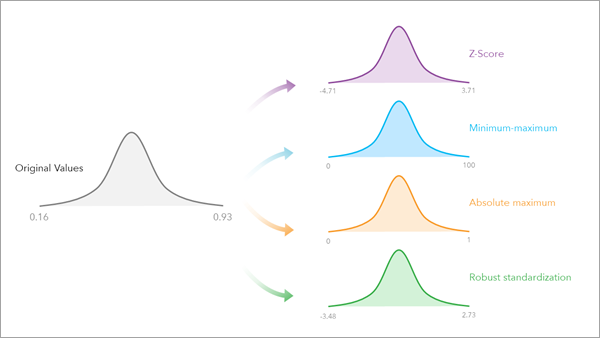## Usage

• There are four standardization methods: Z-Score, Minimum-maximum, Absolute maximum, and Robust standardization.

• The Z-Score method measures the difference between a value and the mean of all values in the field using standard deviations, otherwise known as the standard score.
• Potential application—Assess the significance of a value in relation to the distribution of values in a field. For example, a county's voter participation can be evaluated in the context of other counties across the country, helping identify typical voter participation patterns and counties with significantly high and low voter participation.
• Consideration—This method expects a normal distribution. Consequently, the method is not recommended if the distribution of the data is highly skewed.
• Equation—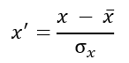, where x' is the standardized value, x is the original value, x̄ is the mean (average), and σx is the standard deviation.
• The Minimum-maximum method preserves the relationships among the original data values while converting the values to a scale between user-specified minimum and maximum values.
• Potential application—A real estate assessor may want to scale characteristics of homes, such as the number of rooms in a house or the age of the house in years to the same scale prior to using these characteristics in a model, such as the Forest-based Classification and Regression tool.
• Consideration—This approach is prone to influence by outliers, or extreme values, in the data.
• Equation—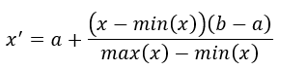, where x' is the standardized value, x is the original value, min(x) is the minimum of the data, max(x) is the maximum of the data, a is the user-specified minimum, and b is the user-specified maximum.
• The Absolute maximum method compares the difference between a value and the maximum absolute value in a distribution by dividing each value by the maximum absolute value in the field.

• Potential application—This method is useful when working with data that has a stable and logical maximum and you want to compare each value to this maximum. For example, the number of votes in a county cannot contain more votes than the number of voting-age people in the county. The county with the highest proportion of votes becomes this maximum, and all other counties are assessed in relation to the absolute maximum voter participation.
• Consideration—The output scale is between -1 and 1. Larger positive values correspond to values close to 1, and larger negative values correspond to values close to -1.
• Equation—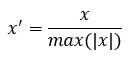, where x' is the standardized value, x is the original value, and max(|x|) is the maximum of the absolute values of the data.

• The Robust standardization method standardizes the values in the specified fields using a robust variant of the z-score. This variant uses median and interquartile range in place of mean and standard deviation.

• Potential application—A real estate assessor is attempting to estimate home values in a city, and an exclusive neighborhood with extremely high home values results in outliers in the data. The assessor uses robust standardization to mitigate the impact of these outliers in the distribution of home values for the city.
• Consideration—With its use of median and interquartile range, this can be an effective method when attempting to mitigate the influence of outliers in the distribution.
• Equation—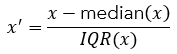, where x' is the standardized value, x is the original value, median(x) is the median of the data, and IQR(x) is the interquartile range of the data.

• If multiple fields are provided, the specified standardization method is applied across all fields.

• The tool modifies the input data and appends the newly created standardized fields to the input table or feature class.

• For each selected field, summary statistics are provided in the geoprocessing message results. These include the maximum, minimum, sum, mean, standard deviation, median, skewness, and kurtosis.

## Parameters

 Label Explanation Data Type Input Table The table containing the field with the values to be standardized. Table View; Raster Layer; Mosaic Layer Field to Standardize The fields containing the values to be standardized. For each field, an output field name can be specified. If an output field name is not provided, the tool will create an output field name using the field name and selected method. Value Table Standardization Method (Optional) Specifies the method to use to standardize the values contained in the specified fields.Z-Score —The standard score, which is the number of standard deviations above or below the mean, is used. The calculation is the Z-Score formula, which calculates the difference between the value and the mean of the values in the column, divided by the standard deviation of the values in the column. This is the default.Minimum-maximum —The values are converted to a scale between the user-specified minimum and maximum values.Absolute maximum —Each value in the column is divided by the maximum absolute value in the column.Robust standardization — A robust variant of the Z-Score formula is used to standardize the values in the specified fields. This variant uses median and interquartile range in place of mean and standard deviation. String Minimum Value (Optional) The value used by the Minimum-maximum method of the Standardization Method parameter to specify the minimum value in the scale of the provided output values. Double Maximum Value (Optional) The value used by the Minimum-maximum method of the Standardization Method parameter to specify the maximum value in the scale of the provided output values. Double

### Derived Output

 Label Explanation Data Type Updated Input Table The table that contains the new encoded fields. Table View

`arcpy.management.StandardizeField(in_table, fields, {method}, {min_value}, {max_value})`
 Name Explanation Data Type in_table The table containing the field with the values to be standardized. Table View; Raster Layer; Mosaic Layer fields[[input_field, output_field],...] The fields containing the values to be standardized. For each field, an output field name can be specified. If an output field name is not provided, the tool will create an output field name using the field name and selected method. Value Table method(Optional) Specifies the method to use to standardize the values contained in the specified fields.Z-SCORE —The standard score, which is the number of standard deviations above or below the mean, is used. The calculation is the Z-Score formula, which calculates the difference between the value and the mean of the values in the column, divided by the standard deviation of the values in the column. This is the default.MIN-MAX —The values are converted to a scale between the user-specified minimum and maximum values.MAXABS —Each value in the column is divided by the maximum absolute value in the column.ROBUST — A robust variant of the Z-Score formula is used to standardize the values in the specified fields. This variant uses median and interquartile range in place of mean and standard deviation. String min_value(Optional) The value used by the MIN-MAX method of the method parameter to specify the minimum value in the scale of the provided output values. Double max_value(Optional) The value used by the MIN-MAX method of the method parameter to specify the maximum value in the scale of the provided output values. Double

### Derived Output

 Name Explanation Data Type updated_table The table that contains the new encoded fields. Table View

### Code sample

StandardizeField example 1 (Python window)

The following Python window script demonstrates how to use the StandardizeField tool.

``````arcpy.management.StandardizeField("County_VoterTurnout",
"voter_turnout voter_turnout_Z_SCORE", "Z-SCORE")``````
StandardizeField example 2 (stand-alone script)

The following stand-alone script demonstrates how to use the StandardizeField tool.

``````# Import system modules
import arcpy

try:
# Set the workspace and input features.
arcpy.env.workspace = r"C:\\Standardize\\MyData.gdb"
inputFeatures = ”County_VoterTurnout”

# Set the input fields that will be standardized

# Set the standardization method.
method = "ROBUST"

# Run the Standardize Field tool
arcpy.management.StandardizeField(inputFeatures, fields, method)

except arcpy.ExecuteError:
# If an error occurred when running the tool, print the error message.
print(arcpy.GetMessages())``````

## Licensing information

• Basic: Yes
• Standard: Yes